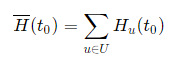Finding a block is a rare and randomly occurring event. However, the pool service needs some reliable method to fairly distribute the value of each found block among pool users and pay them accordingly.

Our reward system ensures that when a new block is found, every user receives a reward proportional to his/her participation in the pool's mining power. Bigger miners receive proportionally more than smaller miners.

## Scoring Hash Rate

User participation in the pool's mining power is measured by scoring hash rate. It reflects how much work the user did in recent hours. You can understand scoring hash rate as an exponential moving average from the hash rate of all users' mining devices connected to the pool (see EMA on wikipedia). The averaging smooths out short hash rate drops or increases and makes rewards calculation more stable and fair (the exact mathematical definition is provided lower).

For example, if your scoring hash rate is 1% of the whole pool's scoring hash rate at the time when a block is found, you receive 1% of the block value for that block. It is that simple.

The pool calculates your reward for every found block as follows: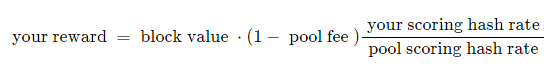A block value consists of the block reward and the fees from transactions contained in the block.

Scoring hash rate used for the calculation is the hash rate from time when a particular block was found. Since it is calculated as an exponential moving average, it reflects some mining history before the actual block find.

Pool fees for all coins are listed in the Rewards & Payouts section.

The chart below shows how the scoring hash rate rises and declines at time when you start or stop mining (effective hash rate is blue, scoring hash rate is gold):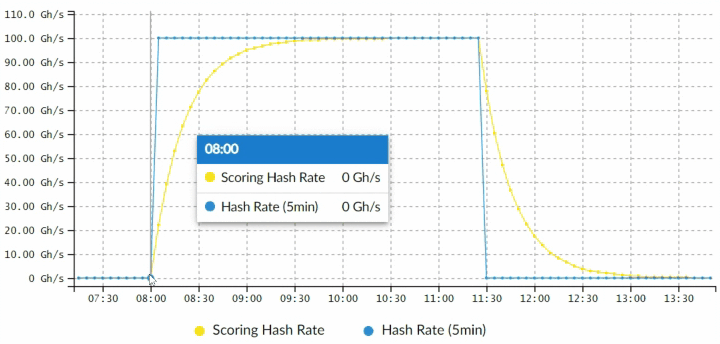The chart would look much more like the one below with a real miner. The reason is that miner's effective hash rate changes in time: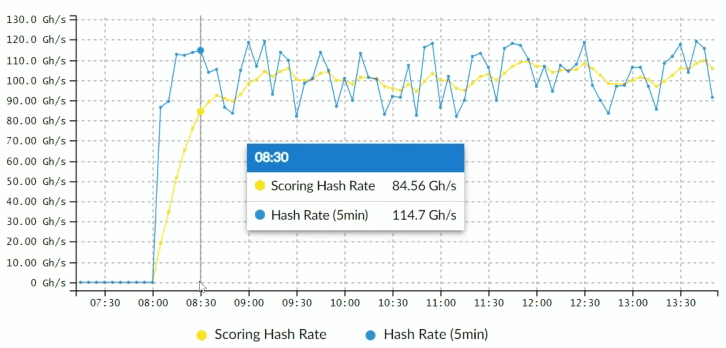## Important Facts about the Method

Important properties of using scoring hash rate for rewards calculation:

You can literally see and understand at any instant how the hash rate is changing and what reward you can expect.

Rewards are fully independent from rounds. The only important thing is the scoring hash rate.

When you start mining, it takes roughly 1.5 hours until it reaches your actual hash rate and then it becomes stable.

It is stable during the time when your miners are connected and working.

When you stop mining the scoring hash rate starts to fall down. It also takes approximately 1.5 hours until it it reaches zero again.

When another miner connects to the pool, your scoring hash rate will stay the same but the pool's hash rate will increase proportionally. As a result, you will receive a smaller part of found blocks as a reward, but blocks will be found more frequently because the pool will be stronger, so your overall earnings should even out. Althernatively, when some other miner disconnects, the opposite will happen. You'll receive a larger portion of the block value, but blocks will be found less frequently. Statistically, there is no difference for you in longer term.

## The Math Behind the Scenes

Let's start with the basic concepts and definitions to describe how exactly rewards and other values presented by the pool are computed. Gradually, we will show more interesting stuff.

### Shares

When a miner connects to the pool it receives a computational task to be solved - it computes hash values with certain properties. Hashes satisfying the task requirements are sent back to the pool and are used as a proof of miner's work. The quantity of miner's work is registered in units called shares. If a hash (proof of work) with difficulty d is submitted by a miner then d shares are accounted by the pool.

Whenever a new share is registered by the system, a timestamp of the event is recorded and used for the share's valuation later.

For the purposes of this text, we will treat each share s as if it was submitted separately as a proof of work with difficulty 1. This approach leads numerically to the same results, however, the formulas are less complex.

### Time handling

Let τ" s="s be a function which gives us a time when some share was submitted (or when a block b was found). The time is measured in seconds from some stable point in time (e.g. start of the pool operation). The time value is only being used for measuring how much time has elapsed between two events. For example, the time between the submission of a share and some point in time t0, written as τ(s) − t0, is a key part of shares valuation.

### Shares valuation - score

A value of each share (for rewards calculation) decreases in time - younger shares are valued higher then older shares. This motivates users to mine with the pool steadily, without switching between pools. Once a user disconnects from the pool, his shares start to lose value.

The exact share value at a given moment in time is defined by scoring function c. It calculates score of a share s at time t0 as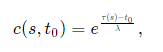where λ is a dimensionless system parameter. λ defines how fast the score assigned to a share declines in time. The greater λ is, the slower old shares loose their value in comparison to new shares. Currently, the parameter λ is set to 1200.

(For users who were familiar with our scoring system presented many years ago, one of the key differences now is that the score is not related to the beginning of the round, but is defined dynamically in relation to any given point in time t0. The change is subtle but has quite significant consequences, because it fully eliminates the concept of rounds from rewards calculation.)

Let's continue. Let U be a set of all pool users and Su(t0) = {s0, s1... } be a set of all valid shares submitted by user U until time t0. Written more formally the following holds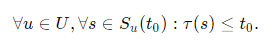Then score for some user U at time t0 is calculated as total score of all his/her shares from time t0, submitted at t0 or earlier: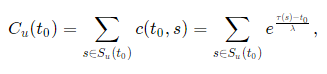Technically, the pool computes the score for each worker separately but the math is analogous.

The score of the whole pool is a sum of the score of all users in the given time together: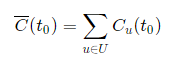\overline C

### Rewards Calculation

When a block b is found at time τ(b) and its value is finally known - V(b), the pool can calculate reward Ru for each user u as follows (f stands for a pool fee):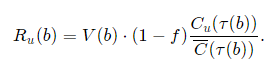For those who really love math equations the full rewards formula for continuous scoring system is: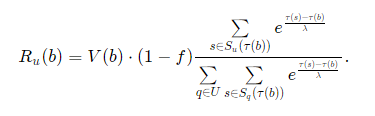### Other values

When you look at your Mining Overview page you will find other values calculated for you by the pool. They are defined as follows. Estimated reward for user u and time t0 is calculated exactly as if a block was found at that time but instead of block value (which is not known yet) we use 6.25 BTC: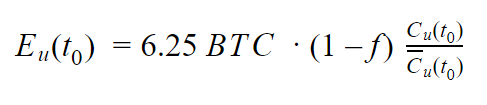User's contribution to the pool (in %) is defined as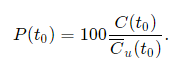And finally the scoring hash rate H for user u and time t0 is internally not calculated by hash rate averaging, but directly from shares using scoring function (result is in h/s):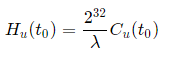The pool's scoring hash rate is not surprisingly defined as all user scoring hash rates together: